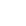Sediment is a mineral or organic material which gets transported by an external weathering agent from its original site and is then deposited to a different place. It is obtained as fragments of rocks which get broken down by mechanical means such as weathering or chemical reactions. The particles can vary from very coarse to very fine depending upon their sizes. The particle size of sediment can provide valuable information about its origin, nature and behaviour. In order to generate information from the particles, instead of determining the size of each grain separately the technique of sub-sampling is brought into practice. For sub-sampling, the representative sediment is run through a set of sieves. Therefore, sample subset gets divided into different classes in terms of varying in sizes. The following data has been collected from the experiments conducted. The error rate for the experiments is taken at 0.03.

From the table 2, it can be inferred that the sediment mass/gram reaches its highest value of 15.41 when a sieve of size 60 µm is used. On the contrary, the sediment mass/gram is lowest (0.01) for a sieve size of 20. However, the percentage of sediment arrives at its maximum value of 14.1 when the sieve size is 80 and the corresponding value of sediment mass/gm is 2.82. The graph follows a hill-like pattern and their peaks indicate the highest values. As shown in the figure 1 (below), sediment mass/gm and the sediment percentage have two separate peak values.

The cumulate mass/gm varies linearly with the sieve size as shown in the figure 2. It attains the highest value of 74.94 when the sieve size is 100. Figures 2 and 3 contain the graphical comparison of cumulative mass/gm, cumulative percentage as well as the sieve sizes. Also, the variations in sediment mass/gm and the cumulative mass/gm with increasing sieve sizes have also been compared for analysis. This comparison can be found in figure 4.

As it can be seen from the figure above, the sizes of sediment obtained reduce steeply with the rise in sediment number. Size of the sediment attains its maximum value of 2000 µm when the mesh number is 10. When the mesh number is changed to 20, there is a sharp and linear reduction in size. There is another steep reduction in sediment size when a mesh of number 40 is used. However, when the mesh number changes from 60 to 100 the sediment size shows a relatively much lesser degree of reduction.

### Related Free Analysis Essays

Free features

• Free bibliography page
• Free delivery
• Free revision
(within 48h)
• Free formatting
• Free outline

We Guarantee

• Quality research and writing
• MA, BA, and PhD degree writers
• 100% Confidentiality
• 100% Authenticity

Paper format

• 12 point font size
• Double or single spacing
• 300 words/page
• Text aligned left
• One-inch marginsTestimonials

Here is what our happy clients say

You may leave feedback on your completed orders